# Es272 ch4a

Counter-Strike Turkiye
13 Dec 2013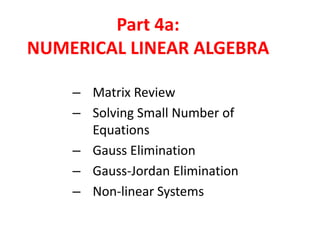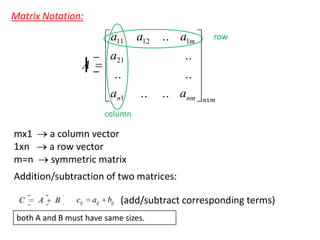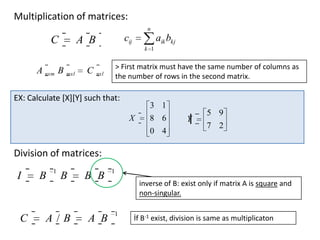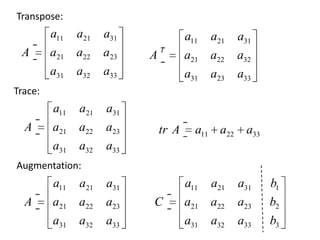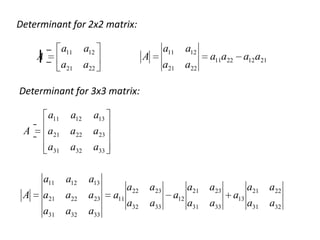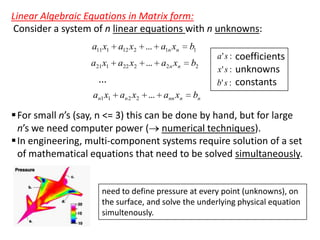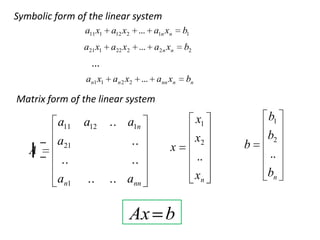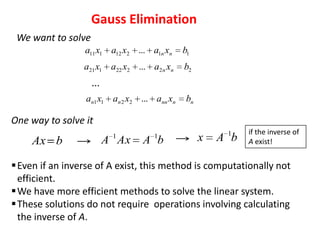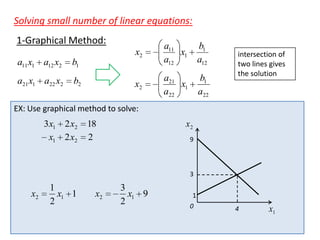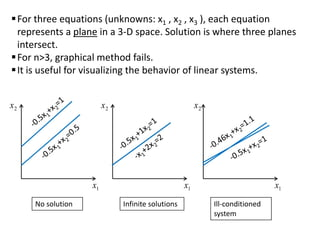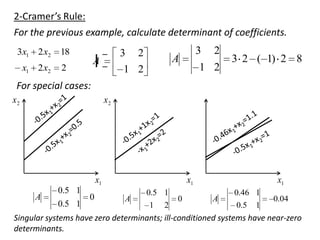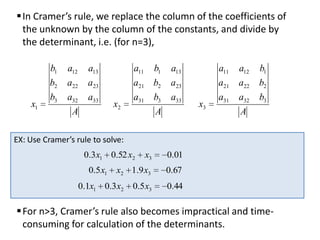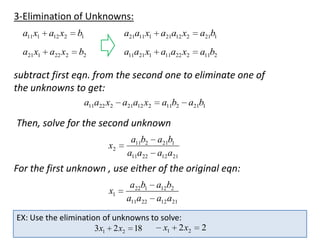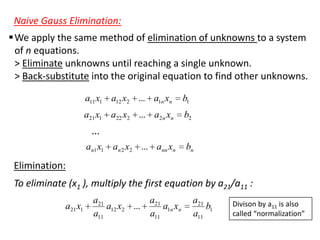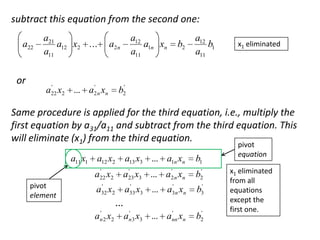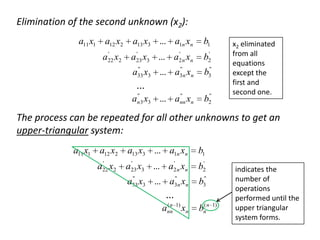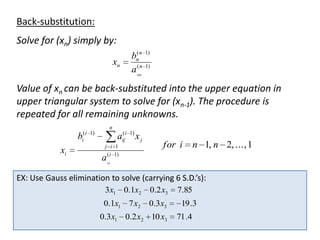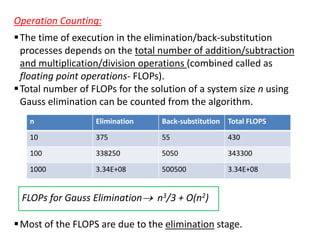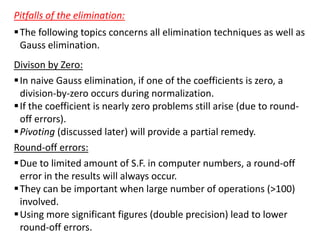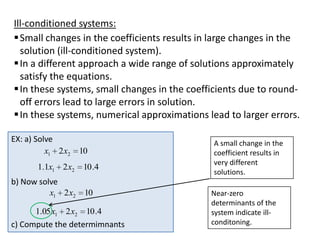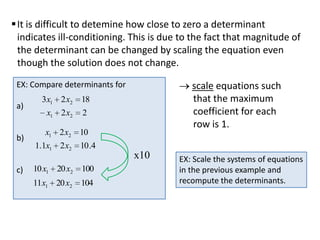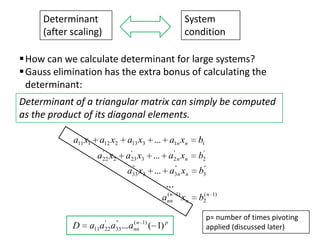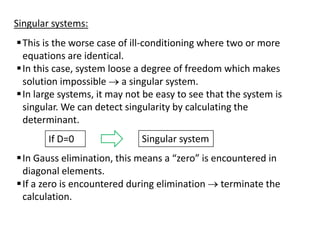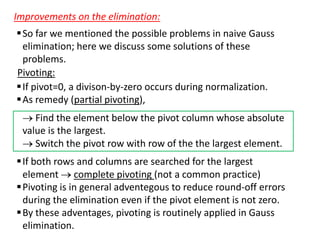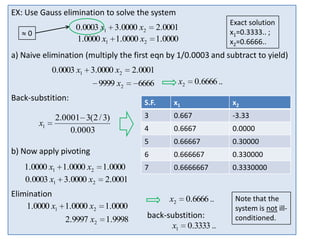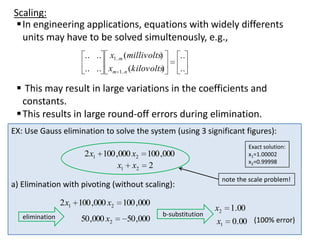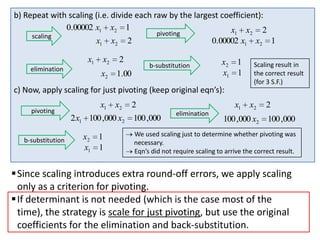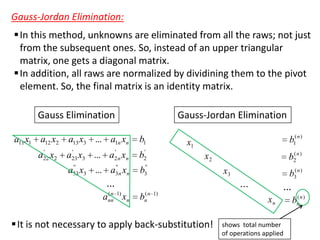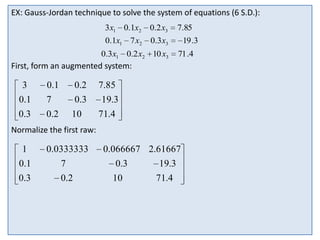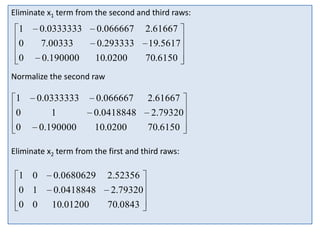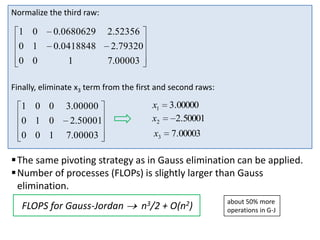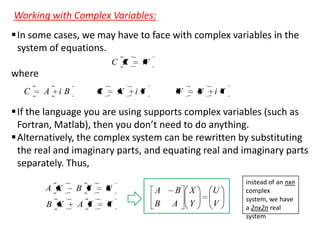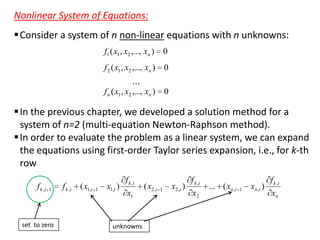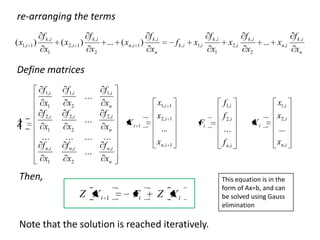1 sur 34

### Es272 ch4a

• 1. Part 4a: NUMERICAL LINEAR ALGEBRA – Matrix Review – Solving Small Number of Equations – Gauss Elimination – Gauss-Jordan Elimination – Non-linear Systems
• 2. Matrix Notation: a11 a21 .. A a12 .. a1m .. .. an1 .. .. anm row nxm column mx1 1xn m=n a column vector a row vector symmetric matrix Addition/subtraction of two matrices: C A B cij aij bij (add/subtract corresponding terms) both A and B must have same sizes.
• 3. Multiplication of matrices: n C cij A B aik bkj k 1 A nxm B mxl C > First matrix must have the same number of columns as the number of rows in the second matrix. nxl EX: Calculate [X][Y] such that: 3 1 X 8 6 0 4 Y 5 9 7 2 Division of matrices: I C B 1 B B B A/B A B 1 inverse of B: exist only if matrix A is square and non-singular. 1 İf B-1 exist, division is same as multiplicaton
• 5. Determinant for 2x2 matrix: a11 A a12 a11 A a21 a22 a12 a21 a22 a11a22 a12 a21 Determinant for 3x3 matrix: a11 a13 a21 a22 a23 a31 A a12 a32 a33 a11 A a12 a13 a21 a22 a23 a31 a32 a33 a11 a22 a23 a32 a33 a12 a21 a23 a31 a33 a13 a21 a22 a31 a32
• 6. Linear Algebraic Equations in Matrix form: Consider a system of n linear equations with n unknowns: a11 x1 a12 x2 ... a1n xn b1 a21 x1 a22 x2 ... a2 n xn b2 ... an1 x1 an 2 x2 ... ann xn a ' s : coefficients x ' s : unknowns b' s : constants bn  For small n’s (say, n <= 3) this can be done by hand, but for large n’s we need computer power ( numerical techniques).  In engineering, multi-component systems require solution of a set of mathematical equations that need to be solved simultaneously. need to define pressure at every point (unknowns), on the surface, and solve the underlying physical equation simultenously.
• 7. Symbolic form of the linear system a11 x1 a12 x2 ... a1n xn b1 a21 x1 a22 x2 ... a2 n xn b2 ... an1 x1 an 2 x2 ... ann xn bn Matrix form of the linear system a11 A a12 a21 .. an1 .. a1n .. .. .. x .. ann Ax b x1 x2 .. xn b b1 b2 .. bn
• 8. Gauss Elimination We want to solve a11 x1 a12 x2 ... a1n xn b1 a21 x1 a22 x2 ... a2 n xn b2 ... an1 x1 an 2 x2 ... ann xn bn One way to solve it Ax b 1 A Ax 1 A b x 1 A b if the inverse of A exist!  Even if an inverse of A exist, this method is computationally not efficient.  We have more efficient methods to solve the linear system.  These solutions do not require operations involving calculating the inverse of A.
• 9. Solving small number of linear equations: 1-Graphical Method: x2 a11 x1 a12 x2 x2 b2 b1 a12 a21 x1 a22 b1 a22 b1 a21 x1 a22 x2 a11 x1 a12 intersection of two lines gives the solution EX: Use graphical method to solve: 3x1 2 x2 x1 2 x2 18 2 x2 9 3 x2 1 x1 1 2 x2 3 x1 9 2 1 0 4 x1
• 10.  For three equations (unknowns: x1 , x2 , x3 ), each equation represents a plane in a 3-D space. Solution is where three planes intersect.  For n>3, graphical method fails.  It is useful for visualizing the behavior of linear systems. x2 x2 x2 x1 No solution x1 Infinite solutions x1 Ill-conditioned system
• 11. 2-Cramer’s Rule: For the previous example, calculate determinant of coefficients. 3x1 2 x2 18 x1 2 x2 2 3 A 2 3 A 2 1 2 1 2 3 2 ( 1) 2 8 For special cases: x2 x2 x1 A 0.5 1 0.5 1 0 x1 A 0.5 1 1 2 0 x1 A 0.46 1 0.5 1 0.04 Singular systems have zero determinants; ill-conditioned systems have near-zero determinants.
• 12.  In Cramer’s rule, we replace the column of the coefficients of the unknown by the column of the constants, and divide by the determinant, i.e. (for n=3), x1 b1 b2 b3 a12 a22 a32 a13 a23 a33 A x2 a11 b1 a21 b2 a31 b3 a13 a23 a33 A x3 a11 a21 a31 a12 a22 a32 b1 b2 b3 A EX: Use Cramer’s rule to solve: 0.3x1 0.52 x2 0.5 x1 x3 0.01 x2 1.9 x3 0.67 0.1x1 0.3x2 0.5 x3 0.44  For n>3, Cramer’s rule also becomes impractical and timeconsuming for calculation of the determinants.
• 13. 3-Elimination of Unknowns: a11 x1 a12 x2 b1 a21a11 x1 a21a12 x2 a21b1 a21 x1 a22 x2 b2 a11a21 x1 a11a22 x2 a11b2 subtract first eqn. from the second one to eliminate one of the unknowns to get: a11a22 x2 a21a12 x2 a11b2 a21b1 Then, solve for the second unknown x2 a11b2 a21b1 a11a22 a12 a21 For the first unknown , use either of the original eqn: x1 a22b1 a12b2 a11a22 a12 a21 EX: Use the elimination of unknowns to solve: x1 2 x2 3x1 2 x2 18 2
• 14. Naive Gauss Elimination:  We apply the same method of elimination of unknowns to a system of n equations. > Eliminate unknowns until reaching a single unknown. > Back-substitute into the original equation to find other unknowns. a11 x1 a12 x2 ... a1n xn b1 a21 x1 a22 x2 ... a2 n xn b2 ... an1 x1 an 2 x2 ... ann xn bn Elimination: To eliminate (x1 ), multiply the first equation by a21/a11 : a21 x1 a21 a12 x2 ... a11 a21 a1n xn a11 a21 b1 a11 Divison by a11 is also called “normalization”
• 15. subtract this equation from the second one: a22 or a21 a12 x2 ... a11 a12 a1n xn a11 a2 n ' ' a22 x2 ... a2 n xn b2 a12 b1 a11 x1 eliminated ' b2 Same procedure is applied for the third equation, i.e., multiply the first equation by a31/a11 and subtract from the third equation. This will eliminate (x1) from the third equation. a11 x1 a12 x2 b1 ' a22 x2 pivot element a13 x3 ... a1n xn ' ' a23 x3 ... a2 n xn ' b2 ' a32 x2 ' ' a33 x3 ... a3n xn b3' ' ' an 3 x3 ... ann xn ' b2 ... ' a n 2 x2 pivot equation x1 eliminated from all equations except the first one.
• 16. Elimination of the second unknown (x2): a11 x1 a12 x2 b1 ' ' a23 x3 ... a2 n xn ' b2 '' ' a33 x3 ... a3' n xn ' a22 x2 a13 x3 ... a1n xn ' b3' x2 eliminated from all equations except the first and second one. ... ' '' an' 3 x3 ... ann xn ' b2' The process can be repeated for all other unknowns to get an upper-triangular system: a11 x1 a12 x2 ' a22 x2 a13 x3 ... a1n xn b1 ' ' a23 x3 ... a2 n xn ' b2 '' ' a33 x3 ... a3' n xn ' b3' ... (n ann 1) xn ( bnn 1) indicates the number of operations performed until the upper triangular system forms.
• 17. Back-substitution: Solve for (xn) simply by: xn ( bnn n a (nn 1) 1) Value of xn can be back-substituted into the upper equation in upper triangular system to solve for (xn-1). The procedure is repeated for all remaining unknowns. n ( i 1) i ( aiji 1) x j b xi j i 1 ( i 1) for i n 1, n 2, ... , 1 a ii EX: Use Gauss elimination to solve (carrying 6 S.D.’s): 3x1 0.1x2 0.2 x3 0.1x1 7 x2 0.3x3 0.3x1 0.2 x2 10 x3 7.85 19 .3 71 .4
• 18. Operation Counting:  The time of execution in the elimination/back-substitution processes depends on the total number of addition/subtraction and multiplication/division operations (combined called as floating point operations- FLOPs).  Total number of FLOPs for the solution of a system size n using Gauss elimination can be counted from the algorithm. n Elimination Back-substitution Total FLOPS 10 375 55 430 100 338250 5050 343300 1000 3.34E+08 500500 3.34E+08 FLOPs for Gauss Elimination n3/3 + O(n2)  Most of the FLOPS are due to the elimination stage.
• 19. Pitfalls of the elimination:  The following topics concerns all elimination techniques as well as Gauss elimination. Divison by Zero:  In naive Gauss elimination, if one of the coefficients is zero, a division-by-zero occurs during normalization.  If the coefficient is nearly zero problems still arise (due to roundoff errors).  Pivoting (discussed later) will provide a partial remedy. Round-off errors:  Due to limited amount of S.F. in computer numbers, a round-off error in the results will always occur.  They can be important when large number of operations (>100) involved.  Using more significant figures (double precision) lead to lower round-off errors.
• 20. Ill-conditioned systems:  Small changes in the coefficients results in large changes in the solution (ill-conditioned system).  In a different approach a wide range of solutions approximately satisfy the equations.  In these systems, small changes in the coefficients due to roundoff errors lead to large errors in solution.  In these systems, numerical approximations lead to larger errors. EX: a) Solve x1 2 x2 10 1.1x1 2 x2 10 .4 b) Now solve x1 2 x2 10 1.05 x1 2 x2 10 .4 c) Compute the determimnants A small change in the coefficient results in very different solutions. Near-zero determinants of the system indicate illconditoning.
• 21.  It is difficult to detemine how close to zero a determinant indicates ill-conditioning. This is due to the fact that magnitude of the determinant can be changed by scaling the equation even though the solution does not change. EX: Compare determinants for a) b) c) 3x1 2 x2 18 x1 2 x2 scale equations such that the maximum coefficient for each row is 1. 2 x1 2 x2 10 1.1x1 2 x2 10 .4 10 x1 20 x2 100 11x1 20 x2 104 x10 EX: Scale the systems of equations in the previous example and recompute the determinants.
• 22. Determinant (after scaling) System condition  How can we calculate determinant for large systems?  Gauss elimination has the extra bonus of calculating the determinant: Determinant of a triangular matrix can simply be computed as the product of its diagonal elements. a11 x1 a12 x2 b1 ' ' a23 x3 ... a2 n xn ' b2 '' ' a33 x3 ... a3' n xn ' a22 x2 a13 x3 ... a1n xn ' b3' ... (n ann 1) xn D ' '' 11 22 33 a a a ...a ( n 1) nn ( 1) p ( b2n 1) p= number of times pivoting applied (discussed later)
• 23. Singular systems:  This is the worse case of ill-conditioning where two or more equations are identical.  In this case, system loose a degree of freedom which makes solution impossible a singular system.  In large systems, it may not be easy to see that the system is singular. We can detect singularity by calculating the determinant. If D=0 Singular system  In Gauss elimination, this means a “zero” is encountered in diagonal elements.  If a zero is encountered during elimination terminate the calculation.
• 24. Improvements on the elimination:  So far we mentioned the possible problems in naive Gauss elimination; here we discuss some solutions of these problems. Pivoting:  If pivot=0, a divison-by-zero occurs during normalization.  As remedy (partial pivoting), Find the element below the pivot column whose absolute value is the largest. Switch the pivot row with row of the the largest element.  If both rows and columns are searched for the largest element complete pivoting (not a common practice)  Pivoting is in general adventegous to reduce round-off errors during the elimination even if the pivot element is not zero.  By these adventages, pivoting is routinely applied in Gauss elimination.
• 25. EX: Use Gauss elimination to solve the system 0.0003 x1 3.0000 x2 1.0000 x1 1.0000 x2 0 Exact solution x1=0.3333.. ; x2=0.6666.. 2.0001 1.0000 a) Naive elimination (multiply the first eqn by 1/0.0003 and subtract to yield) 0.0003 x1 3.0000 x2 2.0001 9999 x2 6666 Back-substition: x2 0.6666 .. b) Now apply pivoting 1.0000 x1 1.0000 x2 1.0000 0.0003 x1 3.0000 x2 2.0001 Elimination 1.0000 x1 1.0000 x2 1.0000 2.9997 x2 1.9998 x1 x2 3 0.667 -3.33 4 0.6667 0.0000 5 x1 2.0001 3(2 / 3) 0.0003 S.F. 0.66667 0.30000 6 0.666667 0.330000 7 0.6666667 0.3330000 x2 0.6666 .. back-substition: x1 0.3333 .. Note that the system is not illconditioned.
• 26. Scaling:  In engineering applications, equations with widely differents units may have to be solved simultenously, e.g., .. .. x1..m (millivolts) ) .. .. xm 1..n (kilovolts .. ..  This may result in large variations in the coefficients and constants.  This results in large round-off errors during elimination. EX: Use Gauss elimination to solve the system (using 3 significant figures): Exact solution: x1=1.00002 x2=0.99998 2 x1 100 ,000 x2 100 ,000 x1 x2 2 note the scale problem! a) Elimination with pivoting (without scaling): 2 x1 100 ,000 x2 elimination 50 ,000 x2 100 ,000 50 ,000 b-substitution x2 1.00 x1 0.00 (100% error)
• 27. b) Repeat with scaling (i.e. divide each raw by the largest coefficient): 0.00002 x1 x2 1 x1 x2 2 pivoting scaling x1 x2 2 0.00002 x1 x2 1 x1 elimination x2 x2 2 b-substitution 1.00 x2 1 x1 1 Scaling result in the correct result (for 3 S.F.) c) Now, apply scaling for just pivoting (keep original eqn’s): pivoting b-substitution x1 x2 2 x1 100 ,000 x2 x2 1 x1 1 2 100 ,000 elimination x1 x2 100 ,000 x2 2 100 ,000 We used scaling just to determine whether pivoting was necessary. Eqn’s did not require scaling to arrive the correct result.  Since scaling introduces extra round-off errors, we apply scaling only as a criterion for pivoting.  If determinant is not needed (which is the case most of the time), the strategy is scale for just pivoting, but use the original coefficients for the elimination and back-substitution.
• 28. Gauss-Jordan Elimination:  In this method, unknowns are eliminated from all the raws; not just from the subsequent ones. So, instead of an upper triangular matrix, one gets a diagonal matrix.  In addition, all raws are normalized by dividining them to the pivot element. So, the final matrix is an identity matrix. Gauss Elimination a11 x1 a12 x2 Gauss-Jordan Elimination b1 ' ' a23 x3 ... a2 n xn ' b2 '' ' a33 x3 ... a3' n xn ' a22 x2 a13 x3 ... a1n xn b1( n ) b3'' x1 ... a ( b2 n ) x2 x3 b3( n ) ... ( n 1) nn n x ( n 1) n b  It is not necessary to apply back-substitution! ... xn shows total number of operations applied (n bn )
• 29. EX: Gauss-Jordan technique to solve the system of equations (6 S.D.): 3x1 0.1x2 0.2 x3 0.1x1 7 x2 0.3x3 0.3x1 0.2 x2 10 x3 7.85 19 .3 71 .4 First, form an augmented system: 3 0.1 0.2 0 .1 7 0.3 0. 3 0.2 10 7.85 19.3 71.4 Normalize the first raw: 1 0.0333333 0.066667 2.61667 0. 1 7 0.3 0.3 0.2 10 19.3 71.4
• 30. Eliminate x1 term from the second and third raws: 1 0.0333333 0.066667 2.61667 0 7.00333 0.293333 19.5617 0 0.190000 10.0200 70.6150 Normalize the second raw 1 0.0333333 0 0 1 0.190000 0.066667 0.0418848 10.0200 2.61667 2.79320 70.6150 Eliminate x2 term from the first and third raws: 1 0 0.0680629 0 1 0.0418848 0 0 10.01200 2.52356 2.79320 70.0843
• 31. Normalize the third raw: 1 0 0.0680629 0 1 0.0418848 0 0 1 2.52356 2.79320 7.00003 Finally, eliminate x3 term from the first and second raws: 1 0 0 0 1 0 0 0 1 3.00000 2.50001 7.00003 x1 x2 3.00000 2.50001 x3 7.00003  The same pivoting strategy as in Gauss elimination can be applied.  Number of processes (FLOPs) is slightly larger than Gauss elimination. FLOPS for Gauss-Jordan n3/2 + O(n2) about 50% more operations in G-J
• 32. Working with Complex Variables:  In some cases, we may have to face with complex variables in the system of equations. C Z W where C A iB Z X iY W U iV  If the language you are using supports complex variables (such as Fortran, Matlab), then you don’t need to do anything.  Alternatively, the complex system can be rewritten by substituting the real and imaginary parts, and equating real and imaginary parts separately. Thus, A X B Y U A B X A Y V B B X A Y U V instead of an nxn complex system, we have a 2nx2n real system
• 33. Nonlinear System of Equations:  Consider a system of n non-linear equations with n unknowns: f1 ( x1 , x2 ,..., xn ) 0 f 2 ( x1 , x2 ,..., xn ) 0 ... f n ( x1 , x2 ,..., xn ) 0  In the previous chapter, we developed a solution method for a system of n=2 (multi-equation Newton-Raphson method).  In order to evaluate the problem as a linear system, we can expand the equations using first-order Taylor series expansion, i.e., for k-th row f k ,i 1 set to zero f k ,i ( x1,i 1 x1,i ) f k ,i x1 unknowns ( x2 ,i 1 x2 ,i ) f k ,i x2 ... ( xn ,i 1 xn ,i ) f k ,i xn
• 34. re-arranging the terms ( x1,i 1 ) f k ,i x1 f k ,i ( x2 ,i 1 ) x2 ... ( xn ,i 1 ) f k ,i xn f k ,i x1,i f k ,i x1 x2 ,i f k ,i x2 ... xn ,i Define matrices f1,i x1 f 2 ,i x2 f 2 ,i x1 ... f n ,i x2 ... f n ,i x1 Z f1,i x2 ... ... ... ... f1,i xn f 2 ,i xn ... f n ,i Xi 1 x1,i 1 x2 ,i 1 ... x n ,i 1 Fi ... f n ,i Xi x1,i x 2 ,i ... x n ,i xn Then, Z Xi f1,i f 2 ,i 1 Fi Z Xi Note that the solution is reached iteratively. This equation is in the form of Ax=b, and can be solved using Gauss elimination f k ,i xn